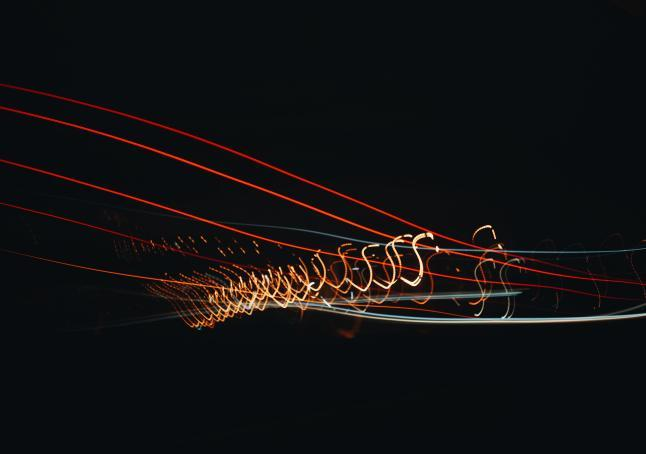Python的matplotlib和seaborn是非常好用的绘图库。但它们创建的都是静态图像，难以通过动态、美观的方式描述数据值的变化。如果你的下一次演示或者下一篇博客文章，能用动态图形展示数据的发展，该有多好？更妙的是，你可以继续使用matplotlib、seaborn或者其他你喜欢用的库。

``````import numpy as np
import pandas as pd
import seaborn as sns
import matplotlib
import matplotlib.pyplot as plt
import matplotlib.animation as animation``````

``````overdoses = pd.read_excel('overdose_data_1999-2015.xls',sheetname='Online',skiprows =6)

def get_data(table,rownum,title):
data = pd.DataFrame(table.loc[rownum][2:]).astype(float)
data.columns = {title}
return data``````

``````%matplotlib notebook
title = 'Heroin Overdoses'
d = get_data(overdoses,18,title)
x = np.array(d.index)
y = np.array(d['Heroin Overdoses'])
overdose = pd.DataFrame(y,x)
#XN,YN = augment(x,y,10)
#augmented = pd.DataFrame(YN,XN)
overdose.columns = {title}``````

``````Writer = animation.writers['ffmpeg']

``````fig = plt.figure(figsize=(10,6))
plt.xlim(1999, 2016)
plt.ylim(np.min(overdose), np.max(overdose))
plt.xlabel('Year',fontsize=20)
plt.ylabel(title,fontsize=20)
plt.title('Heroin Overdoses per Year',fontsize=20)``````

``````def animate(i):
data = overdose.iloc[:int(i+1)]  # 选定数据范围
p = sns.lineplot(x=data.index, y=data[title], data=data, color="r")
p.tick_params(labelsize=17)
plt.setp(p.lines,linewidth=7)``````

``ani = matplotlib.animation.FuncAnimation(fig, animate, frames=17, repeat=True)``

``ani.save('HeroinOverdosesJumpy.mp4', writer=writer)``

``````def augment(xold,yold,numsteps):
xnew = []
ynew = []
for i in range(len(xold)-1):
difX = xold[i+1]-xold[i]
stepsX = difX/numsteps
difY = yold[i+1]-yold[i]
stepsY = difY/numsteps
for s in range(numsteps):
xnew = np.append(xnew,xold[i]+s*stepsX)
ynew = np.append(ynew,yold[i]+s*stepsY)
return xnew,ynew``````

``````def smoothListGaussian(listin,strippedXs=False,degree=5):
window=degree*2-1
weight=np.array([1.0]*window)
weightGauss=[]
for i in range(window):
i=i-degree+1
frac=i/float(window)
gauss=1/(np.exp((4*(frac))**2))
weightGauss.append(gauss)
weight=np.array(weightGauss)*weight
smoothed=[0.0]*(len(listin)-window)
for i in range(len(smoothed)):        smoothed[i]=sum(np.array(listin[i:i+window])*weight)/sum(weight)
return smoothe ``````

``sns.set(rc={'axes.facecolor':'lightgrey', 'figure.facecolor':'lightgrey','figure.edgecolor':'black','axes.grid':False})``